# Three-Cornered Deltoids

[This is the third and final post in this series on triangle geometry. See the previous posts on Morley’s theorem and the 9-point circle.]

For our final exploration in this series, let’s again begin with our triangle ABC and a point P on the circumcircle of the triangle, i.e., the circle through the three vertices. If we drop P directly onto the three lines of the triangle at right angles, then by coincidence, these three points lie on a single line, called the Simson line of point P.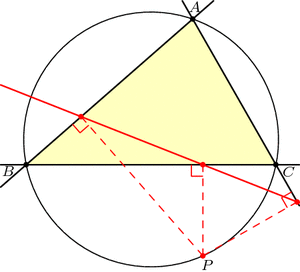Just for fun, let’s draw point Q diametrically opposite from P on the circumcircle, and let’s also look at Q‘s Simson line. How do these two Simson lines interact? Somewhat surprisingly, these lines intersect at right angles. The phenomenal part is that this point of intersection lies on the 9-point circle!Furthermore, as P and Q move around the circumcircle, the intersection of their Simson lines moves around the 9-point circle at twice the speed and in the opposite direction: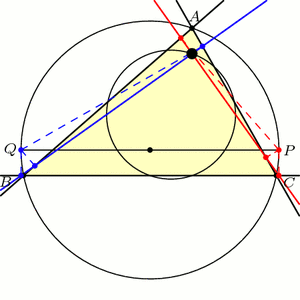This story gets even more unbelievable when we look at how the Simson lines move in this animation. As it turns out, the Simson lines trace a curve in the shape of a deltoid, which is like an equilateral triangle with curved sides. The deltoid traced here is called Steiner’s deltoid.And finally, here’s an incredible fact that ties everything together: If we draw the equilateral triangle around this deltoid, then the edges are parallel to the edges of the equilateral Morley triangle(s).Holy Morley!

### Notes

1. Note that we may have to extend the lines beyond the triangle. []
2. Specifically, a deltoid is the shape that results when you roll a circle inside a circle three-times larger and trace the path of a single point. See Wikipedia:Deltoid_curve for more information. []

# Several Sneaky Circles

In last week’s exploration we uncovered many equilateral triangles hiding in a general triangle ABC. This week we’ll find circles.

Draw any triangle ABC. The line AHA perpendicular to BC is called the altitude though A, with HA at the foot of the altitude. (See the image below.) It can be shown that the three altitudes AHA, BHB, and CHC meet at a single point, H, called the orthocenter of triangle ABC.

Now, let’s put dots at a few important points on our triangle: First, dot the three altitude feet, HA, HB, and HC. Next, dot the midpoints of the sides of the triangle, which we’ll call MA, MB, and MC. Finally, dot the Euler points EA, EB, and EC, which are halfway between H (the orthocenter) and the vertices of our triangle. Today’s first miracle is that all nine of these dots lie on a single circle, aptly named the 9-point circle: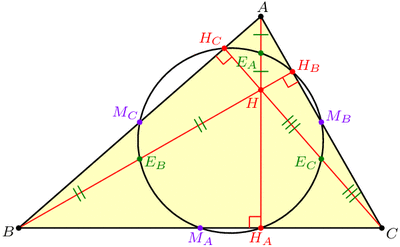The 9-point circle of a triangle is the circle that miraculously passes through the triangle's altitude feet, edge midpoints, and Euler points.

Recall that it only takes three points to determine a circle, so nine on a single circle is very far from coincidence!

Another way to coax a circle out of triangle ABC is to “inscribe” one in the triangle by drawing a circle tangent to the three sides. This circle is called the incircle. Our second miracle is that the incircle and the 9-point circle, which were constructed in very different ways, nevertheless play nicely together: they are tangent! The point where they touch is known as the Feuerbach point of triangle ABC, named after Karl Feuerbach who discovered and proved this difficult fact in 1822.

But there are three other circles that, like the incircle, are tangent to all three lines of the triangle. These “exscribed” circles are called the excircles of triangle ABC, and as luck (or extraversions) would have it, these are also tangent to the 9-point circle:

But wait, there’s more! It turns out that triangles ABC and HBC have the same 9-point circle, and so if we look at the incircle and excircles of triangle HBC, these will also be tangent to our 9-point circle. The same is true for triangles HCA and HAB, so we have found 16 circles all tangent to the nine-point circle!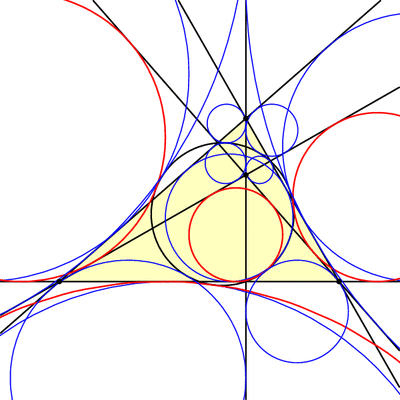The 9-point circle is tangent to the incircle and excircles of triangles ABC, HBC, HCA, and HAB. These four circles for triangle HBC are shown in thick red.

### Notes

1. Recall that we used extraversions last week to go from one Morley triangle to another. Here, extraversions interchange the incircle and excircles. []

# Many Morley Triangles

Given my blog title, how have I gone this long without discussing triangle geometry? I will rectify this gross negligence in the next few weeks. Let’s begin with a seemingly impossible fact due to Frank Morley.

Start with a triangle ABC, with any shape you wish. Now cut its angles into three equal parts, and extend these angle trisectors until they meet in pairs as illustrated below, forming triangle PQR. Morley’s amazing theorem says that this Morley triangle, PQR, will always be equilateral!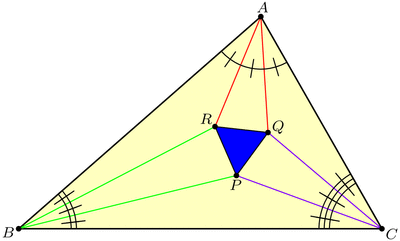Why would this be true? Well, Morley’s theorem tells us that this diagram has three nice 60-degree angles in the middle, but we may suspect that, in fact, all of the angles are nice! This key insight lets us piece together the following argument, where we build up the diagram backwards from its constituent pieces. Draw seven separate “puzzle piece” triangles with angles and side-lengths as shown below, where the original triangle ABC has angles $$3\alpha,3\beta,3\gamma$$ respectively. (Be sure to check that puzzle pieces with these specifications actually exist! Hint: use the Law of Sines.)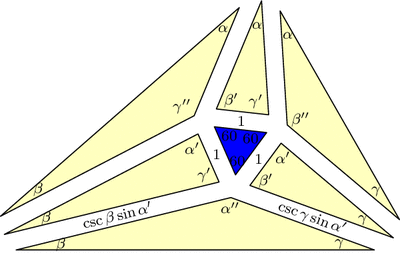Triangle ABC is built out of seven "puzzle pieces" with angles and side-lengths as specified here. In this diagram, $$\alpha^\prime=\alpha+60$$ and $$\alpha^{\prime\prime}=\alpha+120$$, and similarly for $$\beta$$ and $$\gamma$$.

Now, fit the pieces together: all matching edge-lengths are equal (by design), and the angles around vertex P add up to $$60+(\gamma+60)+(\alpha+120)+(\beta+60)=360$$ and similarly for Q and R, so the puzzle fits together into a triangle similar to our original triangle ABC. But now these pieces must make up the Morley configuration, and since we started with an equilateral in the middle, we’re done with the proof!

But there’s more to the Morley story. Let’s push A and C to make them swap positions, dragging the trisectors and Morley triangle along for the ride (a process known as extraversion). We end up using some external angle trisectors instead of only internal ones, but the Morley triangle remains equilateral throughout. This gives us a new equilateral Morley triangle for our original ABC!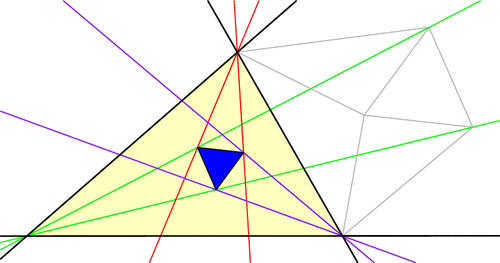In fact, each vertex of ABC has six angle trisectors (three pairs of two), and if you continue applying extraversions you’ll soon uncover that our original triangle ABC has 18 equilateral Morley triangles arranged in a stunning configuration! (Click for larger image.)Here’s a challenge: the diagram above has 27 equilateral triangles, but only 18 of them are Morley triangles (i.e., are made from a pair of trisectors from each vertex). Which ones are they?

### Notes

1. This is a slight modification of a proof by Conway and Doyle. []